## The Cube Roots of Two

Suppose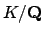is not Galois. Then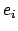,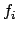, and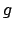are defined for each prime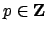, but we need not have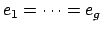or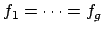. We do still have that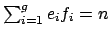, by the Chinese Remainder Theorem.

For example, let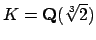. We know that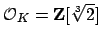. Thus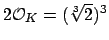, so for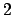we have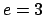and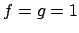. To factor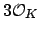, we note that working modulo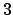we have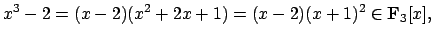so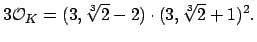Thus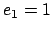,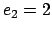,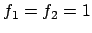, and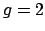. Next, working modulo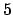we have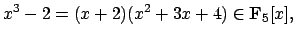and the quadratic factor is irreducible. Thus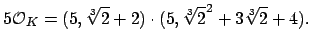Thus here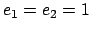,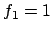,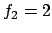, and.

Next we consider what happens in the Galois closure of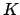. Since the three embeddings of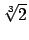in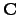are,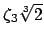, and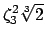, we have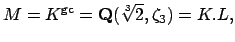where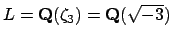, since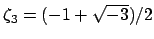is a primitive cube root of unity. The notation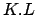means the compositum ofand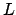'', which is the smallest field generated byand.

Let's figure out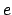,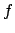, andfor the prime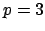relative to the degree six Galois field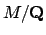by using Theorem 13.2.2 and what we can easily determine aboutand. First, we know that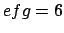. We have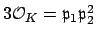, so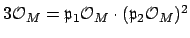, and the prime factors of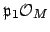are disjoint from the prime factors of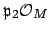. Thus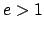is even and also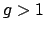. The only possibility for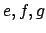satisfying these two conditions is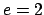,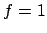,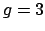, so we conclude that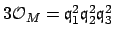without doing any further work, and without actually knowing the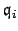explicitly.

Here's another interesting deduction that we can make by hand''. Suppose for the moment that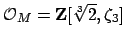(this will turn out to be false). Then the factorization of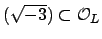in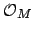would be exactly reflected by the factorization of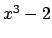in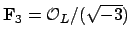. Modulowe have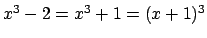, which would imply that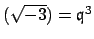for some prime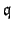of, i.e., that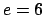and, which is incorrect. Thus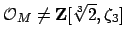. Indeed, this conclusion agrees with the following computation, which asserts that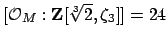:

   > R<x> := PolynomialRing(RationalField());
> K := NumberField(x^3-2);
> L := NumberField(x^2+3);
> M := CompositeFields(K,L);
> O_M := MaximalOrder(M);
> a := M!K.1;
> b := M!L.1;
> O := Order([a,b]);
> Index(O_M,O);
24


William Stein 2004-05-06